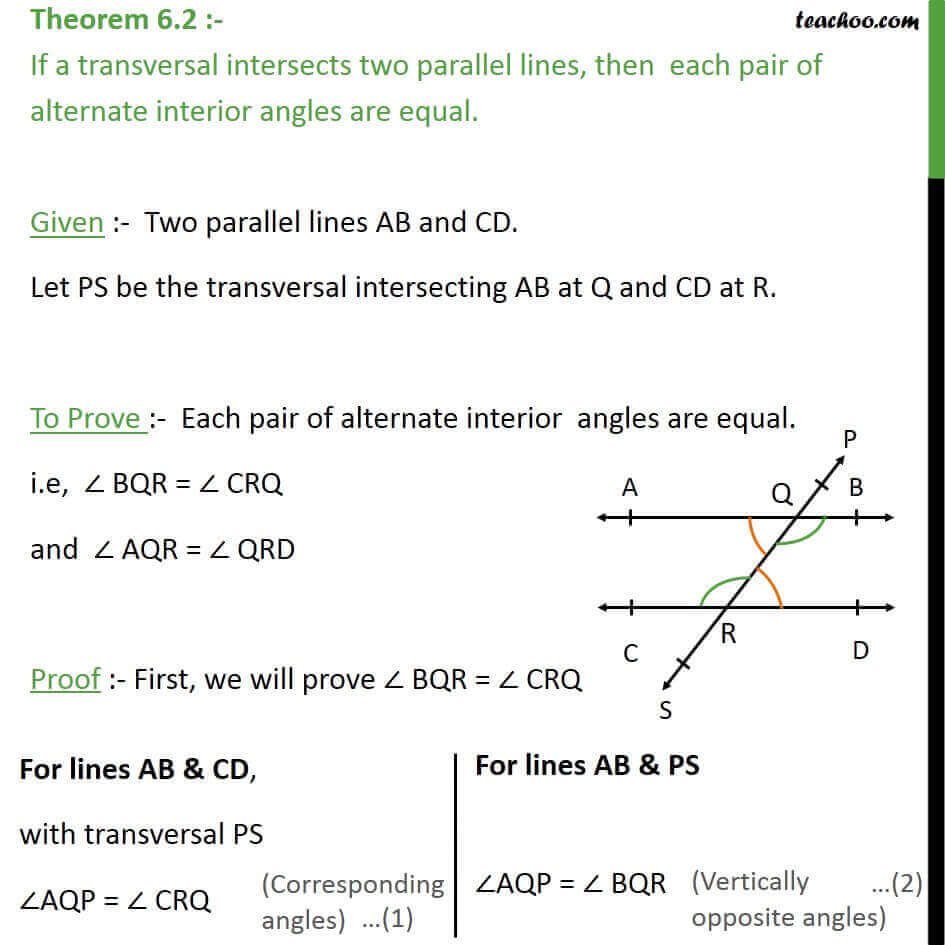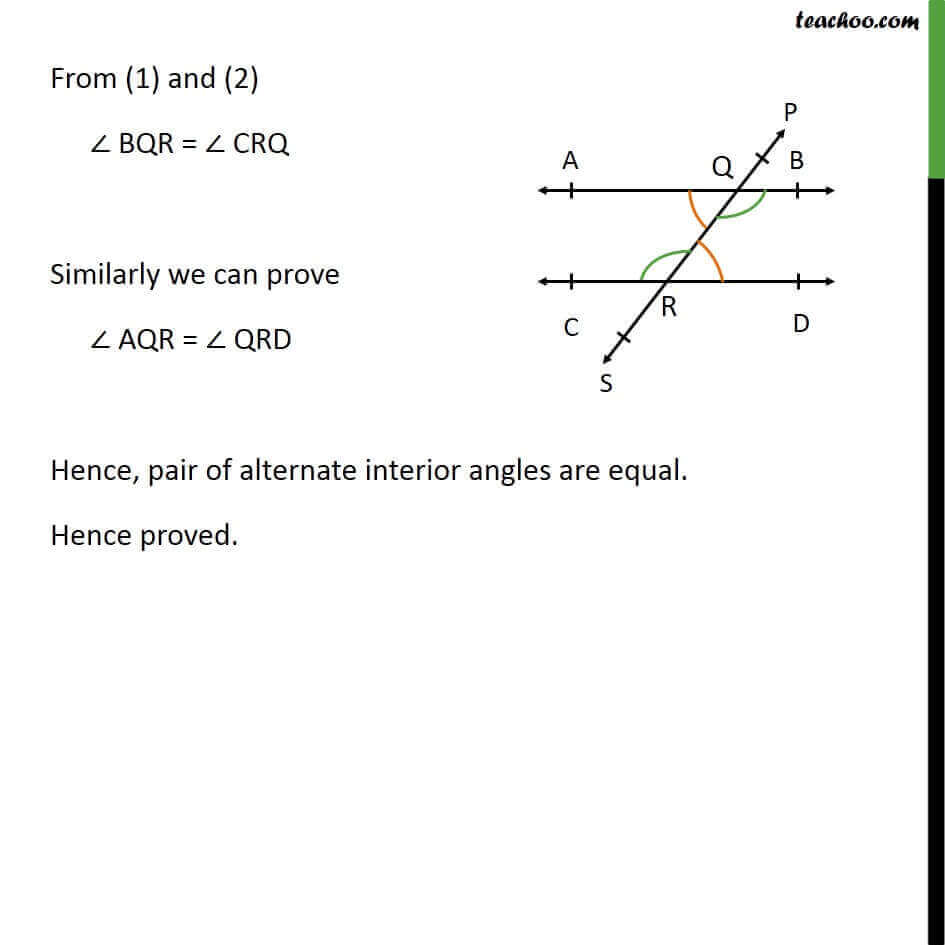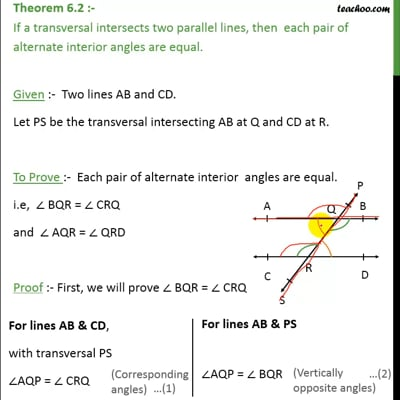Theorems

Chapter 6 Class 9 Lines and Angles
Serial order wiseThis video is only available for Teachoo black users

Introducing your new favourite teacher - Teachoo Black, at only ₹83 per month

### Transcript

Theorem 6.2 :- If a transversal intersects two parallel lines, then each pair of alternate interior angles are equal. Given :- Two parallel lines AB and CD. Let PS be the transversal intersecting AB at Q and CD at R. To Prove :- Each pair of alternate interior angles are equal. i.e, BQR = CRQ and AQR = QRD Proof :- First, we will prove BQR = CRQ From (1) and (2) BQR = CRQ Similarly we can prove AQR = QRD Hence, pair of alternate interior angles are equal. Hence proved.